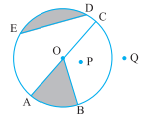# 1. From the figure, identify :(a) the centre of circle (b) three radii(c) a diameter (d) a chord(e) two points in the interior (f) a point in the exterior(g) a sector (h) a segment"

Given:

The figure of a circle is given.

To do:

We have to identify the terms from the given figure.

Solution:

(a) The centre of the circle is $O$.

(b) The three radii are $\overline{OA}, \overline{OB}$ and $\overline{OC}$.

(c) A diameter is $\overline{AC}$.

(d) A chord is $\overline{ED}$.

(e) Two points in the interior are $O$ and $P$

(f) A point in the exterior is point $Q$

(g) A sector is $AOB$(shaded region).

(h) A segment is $ED$(shaded region).